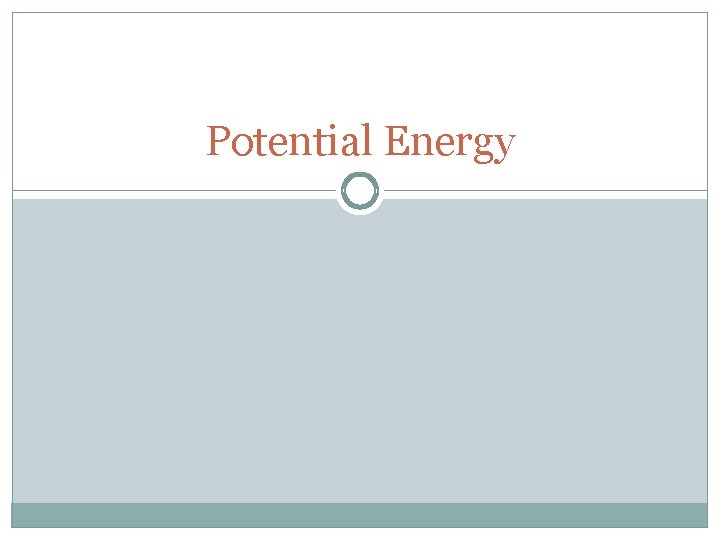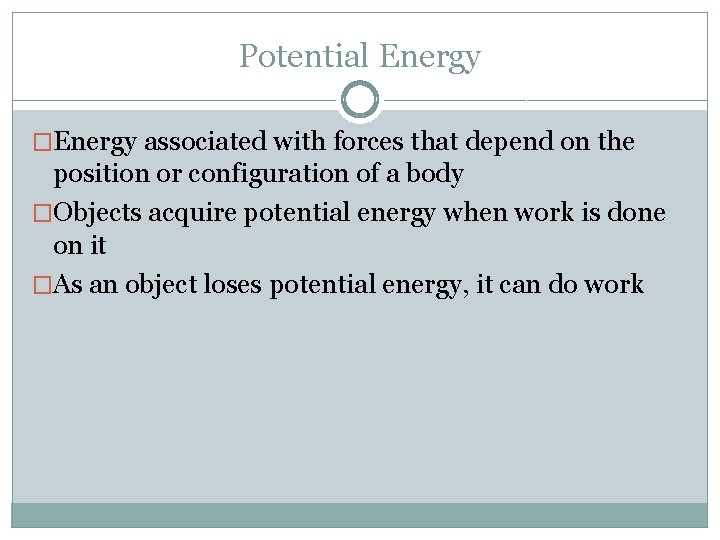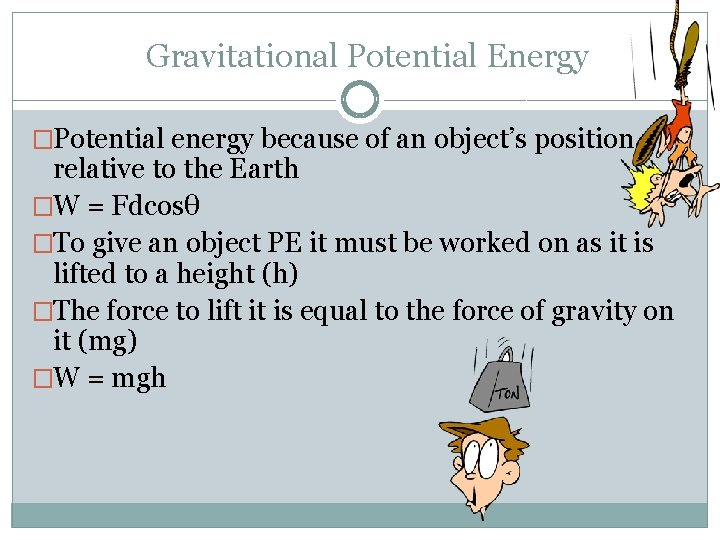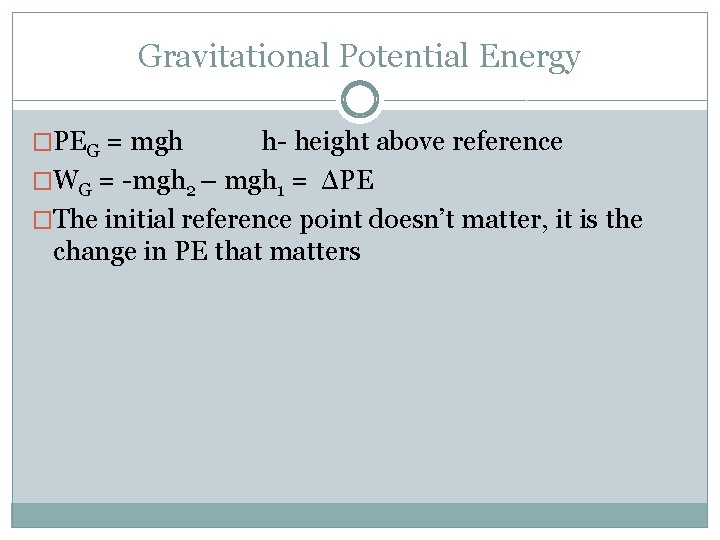Potential Energy Potential Energy Energy associated with forces

• Slides: 7Potential EnergyPotential Energy �Energy associated with forces that depend on the position or configuration of a body �Objects acquire potential energy when work is done on it �As an object loses potential energy, it can do work�Potential energy is the capacity to do work even if not doing work yet �Potential energy can be stored for later use �Equal to the negative of the work done on it to give it this potential energy to begin withGravitational Potential Energy �Potential energy because of an object’s position relative to the Earth �W = Fdcosθ �To give an object PE it must be worked on as it is lifted to a height (h) �The force to lift it is equal to the force of gravity on it (mg) �W = mghGravitational Potential Energy �PEG = mgh h- height above reference �WG = -mgh 2 – mgh 1 = ΔPE �The initial reference point doesn’t matter, it is the change in PE that mattersExample 1 �A 1000 kg roller coaster car moves from pt 1 to 2 and then to 3. A) what is the PE at 2 and 3 relative to 1? B) What is the ΔPE when it goes from 2 to 3? C) Repeat part A and B relative to point 3.Problem How much work does a bricklayer do to carry 30. 2 kg of bricks from the ground up to the third floor (height = 11. 1 m) of a building under construction? What is the gravitational potential energy of the brick-Earth system when the bricklayer reaches the third floor?